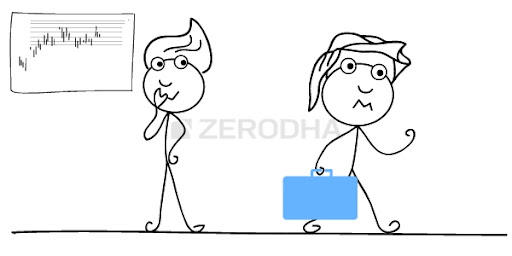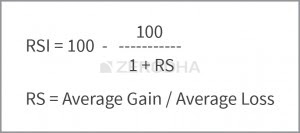## Posts

Showing posts from July, 2018Developed by Etienne Botes and Douglas Siepman, the Vortex Indicator consists of two oscillators that capture positive and negative trend movement. In creating this indicator, Botes and Seipman drew on the work of Welles Wilder and Viktor Schauberger, who is considered the father of implosion technology. Despite a rather involved formula, the indicator is quite straightforward to understand and easy to interpret. A bullish signal triggers when the positive trend indicator crosses above the negative trend indicator or a key level. A bearish signal triggers when the negative trend indicator crosses above the positive trend indicator or a key level. The Vortex Indicator is either above or below these levels, which means it always has a clear bullish or bearish bias. SharpCharts Calculation Calculation of the Vortex Indicator (VTX) can be divided into three parts. First, calculate the positive and negative trend movements based on the highs and lows of the last two periods. Positive tren…Introduction Developed by Tushar Chande and Stanley Kroll, StochRSI is an oscillator that measures the level of RSIrelative to its high-low range over a set time period. StochRSI applies the Stochastics formula to RSI values, instead of price values. This makes it an indicator of an indicator. The result is an oscillator that fluctuates between 0 and 1. In their 1994 book, The New Technical Trader, Chande and Kroll explain that RSI can oscillate between 80 and 20 for extended periods without reaching extreme levels. Notice that 80 and 20 are used for overbought and oversold instead of the more traditional 70 and 30. Traders looking to enter a stock based on an overbought or oversold reading in RSI might find themselves continuously on the sidelines. Chande and Kroll developed StochRSI to increase sensitivity and generate more overbought/oversold signals. CalculationStochRSI = (RSI - Lowest Low RSI) / (Highest High RSI - Lowest Low RSI) StochRSI measures the value of RSI relative to …Pivot Points were originally developed by floor traders in the equity and commodity exchanges. They are calculated based on the high, low and closing prices of previous trading sessions, and they are used by traders to predict support and resistance levels in the current or upcoming session. These support and resistance levels can be used by traders to determine entry and exit points, both for stop-losses and profit taking. [Pivot points are a great way to identify areas of support and resistance, but they work best when combined with other kinds of technical analysis. The Technical Analysis Course on the Investopedia Academy provides a comprehensive overview of both chart patterns and technical indicators, as well as how they can be used to make educated projections and manage risk.] How to Calculate Pivot Points There are several different methods for calculating pivot points, the most common of which is the five-point system. This system uses the previous day's high, low and cl…The Fibonacci Retracements270 The topic on Fibonacci retracements is quite intriguing. To fully understand and appreciate the concept of Fibonacci retracements, one must understand the Fibonacci series. The origins of the Fibonacci series can be traced back to the ancient Indian mathematic scripts, with some claims dating back to 200 BC. However, in the 12th century, Leonardo Pisano Bogollo an Italian mathematician from Pisa, known to his friends as Fibonacci discovered Fibonacci numbers. The Fibonacci series is a sequence of numbers starting from zero arranged in such a way that the value of any number in the series is the sum of the previous two numbers. The Fibonacci sequence is as follows: 0 , 1, 1, 2, 3, 5, 8, 13, 21, 34,  55, 89, 144, 233, 377, 610… Notice the following:
233 = 144 + 89
144 = 89 + 55
89 = 55 +34 Needless to say the series extends to infinity. There are few interesting properties of the Fibonacci series. Divide any number in the series by the previous number; the rat…AroonAbout:Developed by Tushar Chande in 1995, Aroon is an indicator system that determines whether a stock is trending or not and how strong the trend is. “Aroon” means “Dawn’s Early Light” in Sanskrit. Chande chose this name because the indicators are designed to reveal the beginning of a new trend. The Aroon indicators measure the number of periods since price recorded an x-day high or low. There are two separate indicators: Aroon-Up and Aroon-Down. A 25-day Aroon-Up measures the number of days since a 25-day high. A 25-day Aroon-Down measures the number of days since a 25-day low. In this sense, the Aroon indicators are quite different from typical momentum oscillators, which focus on price relative to time. Aroon is unique because it focuses on time relative to price. Chartists can use the Aroon indicators to spot emerging trends, identify consolidations, define correction periods and anticipate reversals. Source: stockcharts.com What should you know? The indicator measures the nu…Relative Strength Index Relative strength Index or just RSI, is a very popular indicator developed by J.Welles Wilder. RSI is a leading momentum indicator which helps in identifying a trend reversal. RSI indicator oscillates between 0 and 100, and based on the latest indicator reading, the expectations on the markets are set. The term “Relative Strength Index” can be a bit misleading as it does not compare the relative strength of two securities, but instead shows the internal strength of the security. RSI is the most popular leading indicator, which gives out strongest signals during the periods of sideways and non trending ranges. The formula to calculate the RSI is as follows: Let us understand this indicator with the help of the following example: Assume the stock is trading at 99 on day 0, with this in perspective; consider the following data points: Sl NoClosing PricePoints GainPoints Lost0110010021022003105300410720051030406100030799010897020910030101055011107201211030131144014…